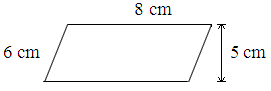# Java Program Calculate Perimeter Of Parallelogram | 3 Ways

Java program to calculate the Perimeter of a parallelogram – In this specific article, we will detail in on the multiple methods to calculate the perimeter of a parallelogram in Java Programming.

Suitable examples and sample outputs are provided for the easy comprehension of the whole scheme of things. The compiler has also been added so that you can execute it yourself.

The methods used in this piece are as follows:

• Using Scanner Class
• Using Command Line Arguments
• Using Static Function

A parallelogram, as we all know, is a two-dimensional quadrilateral figure used in the world of geometry.

A parallelogram, as the name itself suggests, has its opposite sides equal and parallel. The opposite angles are also equal in nature.

A special case where all the angles subtended by a parallelogram are 90 degrees is regarded as a rectangle.As you can see, this is a Parallelogram with the longer side of 8cm and the shorter side of 6cm. Since the opposite sides of a parallelogram are equal, the perimeter can be calculated with this formula:

P = 2(a + b)

Hence, the perimeter of this parallelogram is:

P = 2(8 + 6) = 28cm.

TThus, the various methods to calculate the perimeter of a parallelogram in Java Programming is as follows:

Output:

Output:

Output:
x

## Java Right Arrow Star Pattern Program | Patterns

Java program to print Right Arrow star pattern program. We have written the below print/draw ...